# BooleanTable

Generate a Boolean output signal based on a vector of time instants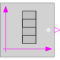# Information

This information is part of the Modelica Standard Library maintained by the Modelica Association.

The Boolean output y is a signal defined by parameter vector table. In the vector time points are stored. The table interpolation has the following properties:

• At every time point, the output y changes its value to the negated value of the previous one.
• Values outside of the table range, are computed by extrapolation according to the setting of parameter extrapolation:
```  extrapolation = 1: Hold the startValue or last value of the table,
if outside of the table scope.
= 2: Extrapolate by using the derivative at the first/last table
points if outside of the table scope.
(This setting is not suitable and triggers an assert.)
= 3: Periodically repeat the table data (periodical function).
= 4: No extrapolation, i.e. extrapolation triggers an error
```
• Via parameter shiftTime the curve defined by the table can be shifted in time. The time instants stored in the table are therefore relative to shiftTime.
• If time < startTime, no interpolation is performed and false is used as ordinate value for the output.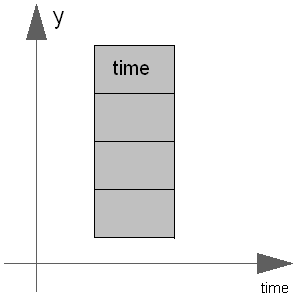The precise semantics is:

```  if size(table,1) == 0 then
y = startValue;
else
//            time < table: y = startValue
// table ≤ time < table: y = not startValue
// table ≤ time < table: y = startValue
// table ≤ time < table: y = not startValue
// ...
end if;
```

# Parameters (5)

table Value: {0, 1} Type: Time[:] (s) Description: Vector of time points. At every time point, the output y gets its opposite value (e.g., table={0,1}) Value: false Type: Boolean Description: Start value of y. At time = table, y changes to 'not startValue' Value: Modelica.Blocks.Types.Extrapolation.HoldLastPoint Type: Extrapolation Description: Extrapolation of data outside the definition range Value: -Modelica.Constants.inf Type: Time (s) Description: Output = false for time < startTime Value: 0 Type: Time (s) Description: Shift time of table

# Connectors (1)

 y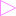Type: BooleanOutput Description: Connector of Boolean output signal

# Components (2)

combiTimeTable realToBoolean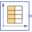Type: CombiTimeTable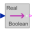Type: RealToBoolean

# Used in Examples (5)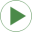ShowLogicalSources Modelica.Blocks.Examples Demonstrates the usage of logical sources together with their diagram animationLogicalNetwork1 Modelica.Blocks.Examples Demonstrates the usage of logical blocksBooleanNetwork1 Modelica.Blocks.Examples Demonstrates the usage of blocks from Modelica.Blocks.MathBooleanInteraction1 Modelica.Blocks.Examples Demonstrates the usage of blocks from Modelica.Blocks.Interaction.ShowCharacteristicThyristors Modelica.Electrical.Analog.Examples Characteristic of ideal thyristors

# Used in Components (3)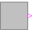RadioButtonSource Modelica.Blocks.Sources Boolean signal source that mimics a radio button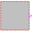RadioButton Modelica.StateGraph.Temporary Button that sets its output to true when pressed and is reset when an element of 'reset' becomes true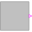RadioButton Modelica.Fluid.Examples.ControlledTankSystem.Utilities Button that sets its output to true when pressed and is reset when an element of 'reset' becomes true